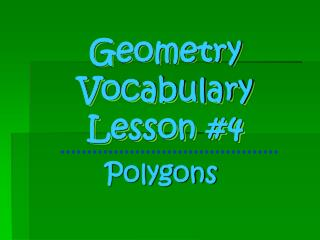Download PresentationGeometry Vocabulary Lesson #4

# Geometry Vocabulary Lesson #4

Download Presentation## Geometry Vocabulary Lesson #4

- - - - - - - - - - - - - - - - - - - - - - - - - - - E N D - - - - - - - - - - - - - - - - - - - - - - - - - - -
##### Presentation Transcript

1. Geometry VocabularyLesson #4 Polygons

2. #27 Polygon • A closed shape with 3 or more straight sides

3. #28 Regular Figure • A polygon with congruent sides and angles

4. #29 Irregular Figure • A polygon that does not have congruent sides and angles

5. Examples:

6. #30 Triangle • A 3-sided polygon • Tri- means 3 • Like tricycle

8. #32 Pentagon • A 5-sided polygon • Penta- means 5 • Like “The Pentagon”

9. #33 Hexagon • A 6-sided polygon • Hexa- means 6

10. STOP #34 Octagon • An 8-sided polygon • Octo- means 8 • Like Octopus

11. #35 Decagon • A 10-sided polygon • Deca- means 10 • Like decade

12. Polygon Prefix # of Sides Triangle Tri- 3 Quadrilateral Quad- 4 Pentagon Penta- 5 Hexagon Hexa- 6 Octagon Octa- 8 Decagon Deca- 10

13. + #36 Perimeter • The distance around a polygon found by adding the lengths of all sides What is the perimeter? 7 ft 5 in 7 ft 5 in 7 ft 5 in 4 ft 4 ft 4 ft 22 ft 10 in

14. #37 Diagonal • A line that goes from one vertex to another, but is NOT an edge